# Generative Art: Part 1

Episode #49 • Mar 4, 2019 • Free Episode

Now that we have made randomness both composable and testable, let’s have a little fun with it! We are going to explore making some complex generative art that is built from simple, composable units.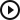Generative Art: Part 1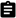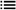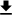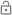This episode is free for everyone.

### Subscribe to Point-Free

Access all past and future episodes when you become a subscriber.

See plans and pricing

### Introduction

In the last two episodes (part 1 and part 2) we finally made the `Gen` type testable. We did this by altering the type so that it wasn’t a function that goes from `Void` to a type `A`, but instead it takes an `inout` parameter of a random number generator and then produces a random `A` value. This meant that we could no longer just hit `run` on our generators and get a random value back, but instead we had to provide a random number generator. This was a good thing because it meant that in production we could supply the system random number generator that comes with Swift, but in tests we could provide a pseudo random number generator that is seedable so that we could produce the same pattern of randomness with each run of the tests.

The best part of all of this was that we achieved testability of the `Gen` type without sacrificing any of the composition that made `Gen` great. We were still allowed to easily `map`, `zip`, `flatMap` generators and even create higher-order functions that return generators in the exact same way we did before make the change to the `Gen` type. The only thing that changed was that at the moment of wanting to run your generator you must supply the random number generator.

The trick we employed to make `Gen` testable, where we fed it an inout parameter, is a universal one, and goes to the heart of how one can manage complex state. We’ll get into that in more detail someday, but today we are going to have some fun with the `Gen` type to show how to break down a complex problem into a bunch of simple pieces that plug together.

We’re going to create some generative art using `Gen` and not only make it predictable for testing, but we are even going to snapshot test it. The generator we are going to create is quite complex, and so it will be really nice to get test coverage on it so that we can refactor and improve it without fear that we are breaking something.

### Generative art

We are going to take some inspiration from the album artwork of the band Joy Division, which has a nice wave form pattern.We’ll build up lots of little generator helpers that will plug together to form mega generator for generating `UIImage`s.

Roughly this is going to be accomplished by using `CoreGraphics` to draw each curve that makes up a line in the graphic. And each line will be made up of lots of little line segments that trace out the curve. We’ll need to come up with some equations to define the curves, as well as some parameters to randomly perturb them, like their position, width, height, and little bit of noise to give it some fuzziness.

Let’s start by defining some constants for our generative artwork:

``````import UIKit

let canvas = CGRect(x: 0, y: 0, width: 600, height: 600)
let mainArea = canvas.insetBy(dx: 130, dy: 100)
let numLines = 80
let numSegments = 80
``````

The `canvas` value determines the over size of the final `UIImage`, whereas the `mainArea` rect determines the area that the artwork will actually be drawn, hence it is inset from the canvas a bit.

We also define constants for the number of lines we are drawing, as well as one for the number of little segments we will draw for each line.

Now, the thing we want to ultimately construct is a generator of `UIImage`s:

``````let image: Gen<UIImage>
``````

If we had that we could simply run the generator to get an image and then plug it into the playground’s live view:

``````import PlaygroundSupport
PlaygroundPage.current.liveView = UIImageView(image: image.run())
``````

And because we care about reproducibility let’s use an LCG:

``````var lcrng = LCRNG(seed: 1)
PlaygroundPage.current.liveView = UIImageView(image: image.run(using: &lcrng))
``````

Now, how can we fill in the `image` generator? Well, let’s kick the can down the road, and let’s suppose we had already created a generator of `CGPath`’s:

``````let paths: Gen<[CGPath]>
``````

When we run this generator we would get an array of `CGPath`s that we could just draw and fill with core graphics very easily. That means we can derive the `image` generator from this `paths` generator by mapping on it. It could look something like:

``````let image = paths.map { paths in
return UIGraphicsImageRenderer(bounds: canvas).image { ctx in
let ctx = ctx.cgContext

ctx.setFillColor(UIColor.black.cgColor)
ctx.fill(canvas)

ctx.setLineWidth(1.2)
ctx.setStrokeColor(UIColor.white.cgColor)

paths.forEach {
ctx.drawPath(using: .fillStroke)
}
}
}
``````

It does the following:

• We `map` on the generator of arrays of paths so that we can transform the paths into an image.
• In the new generator we create a `UIImage` using the closure based API that is provided a `CGContext` to do our drawing in.
• We start by filling the whole canvas with black.
• Then we customize the stroke we will be drawing with.
• And finally we loop over the paths and draw them all with a stroke and a fill.

So now we are left with constructing this generator for random arrays of paths. We can break this down further into a smaller problem of just creating a single random path, and then from that we should be able to create an array of paths. Now, creating a random path generator needs a bit of customization first. We want to know where we start on the x-axis, where we end, and where is the baseline on the y-axis. And then from that configuration we hope that we can create a random `CGPath`.

Let’s start by putting down the signature:

``````func path(from min: CGFloat, to max: CGFloat, baseline: CGFloat) -> Gen<CGPath> {
fatalError()
}
``````

The majority of our work is going to happen in this function, but for a moment let’s assume it’s implemented and see if it helps us implement our `paths` generator. We want to create a path for each line in the artwork, which means we should start by calculating the baseline y-position of each line. We can do that by using the `stride` free function:

``````stride(from: mainArea.minY, to: mainArea.maxY, by: mainArea.height / CGFloat(numLines))
``````

This is a sequence of `CGFloat`s, one for each y-position of a curve in the artwork. We can `map` on this and apply our `path` function to get an array of generators:

``````stride(from: mainArea.minY, to: mainArea.maxY, by: dy)
.map { y in path(from: mainArea.minX, to: mainArea.maxX, baseline: 7) }
``````

This is nearly what we want. It’s an array of generators of paths, whereas we want a generator of an array of paths. Is there anyway to transform the latter into the former? It’s kind of like we are flipping the generic containers around.

Let’s give it a shot:

``````func collect<A>(_ gens: [Gen<A>]) -> Gen<[A]> {
return Gen<[A]> { rng in
gens.map { gen in gen.run(using: &rng) }
}
}
``````

We can now use this function to “collect” our array of generators to a generator of arrays:

``````let paths = collect(
stride(from: mainArea.minY, to: mainArea.maxY, by: dy)
.map { path(from: mainArea.minX, to: mainArea.maxX, baseline: \$0) }
)
``````

We now have an honest generator of arrays of paths, and so our entire generative art rests on the shoulders of the `path` function.

This function is definitely the hardest part of this entire project, so let’s keep simplifying. Let’s first start by just drawing straight lines across the screen with no randomness at all.

We know we need to return a `Gen` from `path` so we can start there. It’s initializer takes a closure that gives us access to a random number generator:

``````func path(from min: CGFloat, to max: CGFloat, baseline: CGFloat) -> Gen<CGPath> {
return Gen<CGPath> { rng in
fatalError()
}
}
``````

In here is where we do all of our work. To keep things simple we’ll just draw a line from the left side of the screen to the right side:

``````func path(from min: CGFloat, to max: CGFloat, baseline: CGFloat) -> Gen<CGPath> {
return Gen<CGPath> { rng in
let path = CGMutablePath()
path.move(to: CGPoint(x: min, y: baseline))
return path
}
}
``````

And for the first time we’ve actually got something on the screen and it’s kinda cool!Now let’s increase the complexity of this just a bit by not simply drawing a single line from left-to-right, but drawing a whole bunch of little line segments using the `min` and `max` values:

``````func path(from min: CGFloat, to max: CGFloat, baseline: CGFloat) -> Gen<CGPath> {
return Gen<CGPath> { rng in
let path = CGMutablePath()
path.move(to: CGPoint(x: min, y: baseline))
stride(from: min, to: max, by: mainArea.width / CGFloat(numSegments)).forEach { x in
}
return path
}
}
``````

This produces the same image, but now we have a bunch of little line segments that we can try to perturb into some interesting art.

And now it gets down to the interesting part, where we have to come up with a way to draw the curves. Let’s take a quick detour to discuss some interesting mathematics.

### Bump functions

There is a class of functions in math known as “bump functions”. They are simple functions from the real numbers to the real numbers that are basically zero everywhere, but at some point it smoothly goes from 0 to 1, stays at 1 for a bit, and then goes back to 0. Making a little bump!So, how do you construct a bump function? We are going to do this with a bunch of steps, and to visualize it along the way we have a little function graphing helper. You can give `graph` any function `(CGFloat) -> CGFloat` and you will get back a plot of that graph as a `UIImage`:

``````func graph(_ f: (CGFloat) -> CGFloat) -> UIImage
``````

For simplicity we are only graphing the portion of the function from `-1` to `1`. Also, in `CoreGraphics` the origin of a canvas is in the top left corner, and the y-axis increases as you go down the screen, whereas in mathematics we are used to the origin being in the center and the y-axis increasing as you go up. So this `graph` helper has little bit of logic built in to correct for that.

We could graph the identity function just to make sure it works:

``````PlaygroundPage.current.liveView = UIImageView(image: graph { \$0 })
``````It’s just a simple straight line from the bottom-left corner to the top-right corner. We could also try graphing the parabola:

``````PlaygroundPage.current.liveView = UIImageView(image: graph { \$0 * 0 })
``````And finally we could plot a sine curve:

``````PlaygroundPage.current.liveView = UIImageView(image: graph { sin(\$0) })
``````This will help us visualize our bump function as we build it up, because it’s going to take a few steps. We start a very simple, well behaved function:

``````func f(_ x: CGFloat) -> CGFloat {
if x <= 0 { return 0 }
return exp(-1 / x)
}

PlaygroundPage.current.liveView = UIImageView(image: graph({ f(\$0) })
``````

This functions is `0` for all values of `x` less than or equal to `0`, but right after that is `e` to the power of `-1/x`, which causes it to smoothly start to trend up, and then slowly flatten out as `x` gets better, but it never goes higher than `1`.There’s a trick we can employ to turn this into a function that smoothly transitions from 0 to 1 as `x` ranges from 0 to 1:

``````func g(_ x: CGFloat) -> CGFloat {
return f(x) / (f(x) + f(1 - x))
}

PlaygroundPage.current.liveView = UIImageView(image: graph({ g(\$0) })
``````

Now we’re getting somewhere. This functions travels from `(0, 0)` to `(1, 1)` in a smooth fashion.We can make this seem more “bump”-like by plugging `x^2` into the equation, because then it will be symmetric across the y-axis:``````func h(_ x: CGFloat) -> CGFloat {
return g(x * x)
}

PlaygroundPage.current.liveView = UIImageView(image: graph({ h(\$0) })
``````

And finally we can invert the function and shift it so that it looks like a real bump:

``````func bump(_ x: CGFloat) -> CGFloat {
return 1 - h(x)
}

PlaygroundPage.current.liveView = UIImageView(image: graph({ bump(\$0) })
``````Now we have a real bump! We just have to figure out how to parameterize it so that we can control its height, width and positioning.

Let’s start with the easiest part: controlling the height. To do that we just multiply the final result by a factor:

``````func bump(amplitude: CGFloat, _ x: CGFloat) -> CGFloat {
return amplitude * (1 - _g(x * x))
}

PlaygroundPage.current.liveView = UIImageView(image: graph { bump(amplitude: 0.5, \$0) })
``````Next let’s figure out how to move it along the x-axis. This is just a matter of precomposing with a transformation that translates the `x` parameter of the function. Only tricky part here is that to translate we need to actually subtract, not add:``````func bump(amplitude: CGFloat, center: CGFloat, _ x: CGFloat) -> CGFloat {
let x = x - center
return amplitude * (1 - _g(x * x))
}

PlaygroundPage.current.liveView = UIImageView(image: graph { bump(amplitude: 0.5, center: 0.25, \$0) })
``````

And finally, we need a way to control the width of the bump. There are two parameters at work for this. We want to express the point where the bump begins to lift off the x-axis, and then the point the bump levels out at its plateau. It’s a lot more complicated to accomplish this, but this will do the trick:

``````func bump(
amplitude: CGFloat,
center: CGFloat,
plateauSize: CGFloat,
curveSize: CGFloat,
_ x: CGFloat
) -> CGFloat {
let plateauSize = plateauSize / 2
let curveSize = curveSize / 2
let size = plateauSize + curveSize
let x = x - center
return amplitude * (1 - g((x * x - plateauSize * plateauSize) / (size * size - plateauSize * plateauSize)))
}

PlaygroundPage.current.liveView = UIImageView(image: graph { bump(amplitude: 0.5, center: 0, plateauSize: 0, curveSize: 1.5, \$0) })
``````And we now have all the parameters necessary to move and scale this bump function to anywhere we want. This will form the basis of our image.

This is a lot to take in at once, but what’s cool is had you known you were looking for equations of functions that have bumps in them, you would have been able to search Wikipedia or Google for bump functions and you would have been able to code up these functions by translating them to Swift.

### Constructing random paths

Let’s go back to our `path` function for creating generators of random paths, and clear out the body so that we can start fresh:

``````func path(from min: CGFloat, to max: CGFloat, baseline: CGFloat) -> Gen<CGPath> {
let dx = mainArea.width / CGFloat(numSegments)
return Gen<CGPath> { rng in
let path = CGMutablePath()
path.move(to: CGPoint(x: min, y: baseline))
stride(from: min, to: max, by: dx).forEach { x in
// ???
}
return path
}
}
``````

Let’s start by invoking the `bump` function inside the stride, and we’ll just hard code some parameters for now to get a feel for it:

``````func path(from min: CGFloat, to max: CGFloat, baseline: CGFloat) -> Gen<CGPath> {
return Gen<CGPath> { rng in
let path = CGMutablePath()
path.move(to: CGPoint(x: min, y: baseline))
stride(from: min, to: max, by: dx).forEach { x in
let y = bump(
amplitude: 10,
center: canvas.width / 2,
plateauSize: 50,
curveSize: 50,
x
)
path.addLine(to: CGPoint(x: x, y: baseline + y))
}
return path
}
}
``````

Running this and we get something a little weird.Remember how we said `CoreGraphics`’ y-axis points down? Well, our `graph` helper accommodated for that, but now we have to do it ourselves. The fix is easy, just use a negative amplitude:

``````func path(from min: CGFloat, to max: CGFloat, baseline: CGFloat) -> Gen<CGPath> {
return Gen<CGPath> { rng in
let path = CGMutablePath()
path.move(to: CGPoint(x: min, y: baseline))
stride(from: min, to: max, by: dx).forEach { x in
let y = bump(
amplitude: -10,
center: canvas.width / 2,
plateauSize: 50,
curveSize: 50,
x
)
path.addLine(to: CGPoint(x: x, y: baseline + y))
}
return path
}
}
``````

And now we’re getting closer.Next we want to add some randomness to the parameters of this curve. We could generate random values for amplitude, center, plateau size and curve size right in this `forEach` and then pass them along to the `bump` function, but then we would be getting a completely different bump function for each invocation of the `forEach`. We could also just lift the constants we are feeding to `bump` out of the `forEach` and compute them randomly up there.

However, a nicer way would be to just create a random bump function up top, and then use that in the `forEach`. That way we don’t have a bunch of constants floating around whose only purpose is to be fed into the bump function. We can just concentrate on the bump function itself.

So let’s try that. We want something with the following signature:

``````let curve: Gen<(CGFloat) -> CGFloat>
``````

This is already pretty interesting, we are generating random functions. But how can we create this? One way would be to do it from scratch:

``````let curve = Gen<(CGFloat) -> CGFloat> { rng in
{ x in
return ???
}
}
``````

Now we need to compute the parameters. We can use some our `Gen` helpers to do that:

``````let curve = Gen<(CGFloat) -> CGFloat> { rng in
let amplitude = Gen<CGFloat>.float(in: 1...20)
.run(using: &rng)
let center = Gen<CGFloat>.float(in: -60...60).map { \$0 + canvas.width / 2 }
.run(using: &rng)
let plateauSize = Gen<CGFloat>.float(in: 0...60)
.run(using: &rng)
let curveSize = Gen<CGFloat>.float(in: 10...60)
.run(using: &rng)

return { x in
bump(
amplitude: amplitude,
center: center,
plateauSize: plateauSize,
curveSize: curveSize,
x
)
}
}
``````

And we don’t yet have a random curve, we only have a generator for curves. So, to get a curve out of it we need to run it:

``````func path(from min: CGFloat, to max: CGFloat, baseline: CGFloat) -> Gen<CGPath> {
return Gen<CGPath> { rng in
let bump = curve.run(using: &rng)
let path = CGMutablePath()
path.move(to: CGPoint(x: min, y: baseline))
stride(from: min, to: max, by: dx).forEach { x in
let y = bump(x)
path.addLine(to: CGPoint(x: x, y: baseline + y))
}
return path
}
}
``````

And, oops, looks like we forgot to negate the amplitude again.Let’s fix that.

``````let amplitude = Gen<CGFloat>.float(in: -20...(-1))
``````

And now we’re getting somewhere.However, all that work we are doing to get a random curve is obscuring the point of that code. There is something much simpler we can do. We can use `zip`. We’d like to be able to plug `Gen` values directly into the `bump` function, and we’ve seen that the `zip(with:)` function allows us to do precisely that. Only problem is that we don’t want to use a `Gen` value for the first parameter, because that’s the `x` that gets plugged into the function later on. We need to employ a technique we’ve discussed a number of times on this series where we make a function more composable and reusable by moving all the configuration parameters to the front, and currying out the data parameters.

That makes `bump` look like this:

``````func bump(
amplitude: CGFloat,
center: CGFloat,
plateauSize: CGFloat,
curveSize: CGFloat
) -> (CGFloat) -> CGFloat {
return { x in
let plateauSize = plateauSize / 2
let curveSize = curveSize / 2
let size = plateauSize + curveSize
let x = x - center
return amplitude * (1 - g((x * x - plateauSize * plateauSize) / (size * size - plateauSize * plateauSize)))
}
}
``````

And with just a few small changes we can express the random curve as a `zip` of a bunch of generators:

``````let curve = zip(
with: bump(amplitude:center:plateauSize:curveSize:),
Gen<CGFloat>.float(in: 1...20).map { -\$0 },
Gen<CGFloat>.float(in: -60...60).map { \$0 + canvas.width / 2 },
Gen<CGFloat>.float(in: 0...60),
Gen<CGFloat>.float(in: 10...60)
)
``````

And if we run this we get the same result.

### To be continued…

So we’re getting really close to the image we are trying to replicate, but there are a few small things that would make it even better.

• Next we could add a bit of noise to the curves so that they look more ripply and sound-wavey. We could even get fancy and make it so that the noise is less towards the edges of the image, and more intense where the bump takes place.
• Right now our bumps are pretty boring and uniform. What we can do is draw multiple random bumps on each line, maybe even a random number of random bumps, and whenever two bumps overlap we will have their amplitudes add. This will give us some more interesting bumps with more variation.

Accomplishing this will lead us to some very complicated generators, and so once we’ve done that we’ll probably want to add some test coverage to these generators. And that’s exactly what we’ll do now…This episode is free for everyone.

### Subscribe to Point-Free

Access all past and future episodes when you become a subscriber.

See plans and pricing

### Exercises

1. Create a generator `Gen<UIColor>` of colors. Can this be expressed in terms of multiple `Gen<CGFloat>` generators?

2. Create a generator `Gen<CGPath>` of random lines on a canvas. Can this be expressed in terms of multiple `Gen<CGPoint>` generators?

3. Create a generator `Gen<UIImage>` that draws a random number of randomly positioned straight lines on a canvas with random colors. Try to compose this generator out of lots of smaller generators.

4. Change the `bump` function we created in this episode so that it adds a bit of random noise to the curve. To do this you will want to change the signature so that it returns a function `(CGFloat) -> Gen<CGFloat>` instead of just a simple function `(CGFloat) -> CGFloat`. This will allow you to introduce random perturbations into the y-coordinate of the graph.

#### SE-0202: Random Unification

Alejandro Alonso • Friday Sep 8, 2017

This Swift Evolution proposal to create a unified random API, and a secure random API for all platforms, was accepted and implemented in Swift 4.2.

#### Unknown Pleasures – Artwork and packaging

Wikipedia contributors • Wednesday Jan 2, 2019

The artwork used as inspiration in this episode comes from the album cover from the band Joy Division.

#### Bump Function

Wikipedia contributors • Friday Apr 6, 2018

We used “bump functions” in this episode to construct functions that are zero everywhere except in a small region where they smoothly climb to 1 and then plateau. They are useful in mathematics for taking lots of local descriptions of a function and patching them together into a global function.